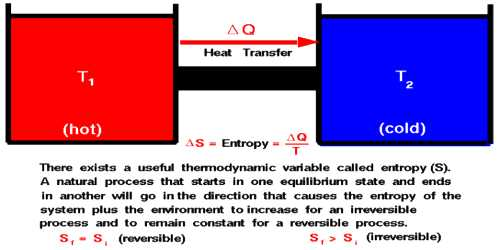Physics

# Formulation of Second Law of Thermodynamics in terms of EntropyFormulation of Second Law of Thermodynamics in terms of Entropy

The thermal property that remains constant in the adiabatic process is called entropy. Alternately, entropy is the physical property of the body that remains constant in an adiabatic process.

According to Clausius, the first law of thermodynamics is as follows:

The total energy of the universe is constant. It is called the conservation principle of energy. Clausius defined the second law of thermodynamics in the following way:

The entropy of the universe is progressively increasing. It can also be called the law of increase of entropy. Naturally, we can define the second law of thermodynamics in terms of entropy in the following way:

All physical or chemical actions take place in such a way that as a result of which entropy of the whole system increases. In a limiting case, the entropy of a reversible process remains unchanged.

The second law of thermodynamics can be stated in terms of entropy. If a reversible process occurs, there is no net change in entropy. In an irreversible process, entropy always increases, so the change in entropy is positive. The total entropy of the universe is continually increasing. In order to define the second law of thermodynamics let us consider the values of the entropy of the initial and final state A and B are respectively SA and SB. So, change of entropy of the system,

SB – SA = ∫AB dQ/T  ….  …. (1)

If the two states A and B are very dale to each other, then we can write dS = dQ/T

then, dQ = T dS … … … (2)

This is the mathematical definition of the second law of thermodynamics.

We know entropy increases in caw of irreversible process and entropy remains constant in the reversible process. Most of the processes in the universe are an irreversible process. So, it can be said that the entropy of the universe is progressively increasing.